Select Question Set:A tube of length L is filled completely with an incompressible liquid of mass M and closed at both ends. The tube is then rotated in a horizontal plane about one of its ends with a uniform angular velocity $\omega$. The force exerted by liquid at the other end is

1.  $\frac{{\mathrm{M\omega }}^{2}\mathrm{L}}{2}$

2.  ${\mathrm{ML\omega }}^{2}$

3.  $\frac{{\mathrm{M\omega }}^{2}\mathrm{L}}{4}$

4.  $\frac{M{L}^{2}{\omega }^{2}}{2}$Subtopic:  Pressure |
57%
To view explanation, please take trial in the course.
NEET 2023 - Target Batch - Aryan Raj Singh
Hints

The radius of a soap bubble is increased from R to 2 R. Work done in this process (T = surface tension) is:

 1 24 πR2T 2 48 πR2T 3 12 πR2T 4 36 πR2TSubtopic:  Surface Tension |
60%
From NCERT
To view explanation, please take trial in the course.
NEET 2023 - Target Batch - Aryan Raj Singh
Hints
To view explanation, please take trial in the course.
NEET 2023 - Target Batch - Aryan Raj Singh

The flow rate from a tap of diameter 1.25 cm is 3 lit/min. The coefficient of viscosity of water is ${10}^{-3}$ Pas. The nature of flow is :

1. Turbulent

2. Laminar

3. Neither laminar nor turbulentSubtopic:  Types of Flows |
53%
From NCERT
To view explanation, please take trial in the course.
NEET 2023 - Target Batch - Aryan Raj Singh
Hints
To view explanation, please take trial in the course.
NEET 2023 - Target Batch - Aryan Raj Singh

A small spherical solid ball is dropped in a viscous liquid. Its journey in the liquid is best described in the figure by :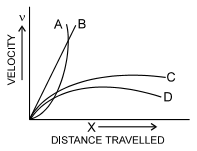1.  Curve A

2.  Curve B

3.  Curve C

4.  Curve DSubtopic:  Stokes' Law |
69%
From NCERT
To view explanation, please take trial in the course.
NEET 2023 - Target Batch - Aryan Raj Singh
Hints
To view explanation, please take trial in the course.
NEET 2023 - Target Batch - Aryan Raj Singh

Water flows through a frictionless duct with a cross-section varying as shown in the figure. Pressure p at points along the axis is represented by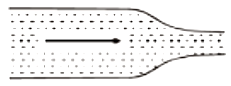1.2.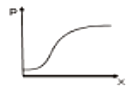3.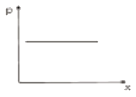4.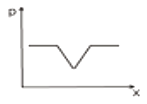Subtopic:  Equation of Continuity |
55%
From NCERT
To view explanation, please take trial in the course.
NEET 2023 - Target Batch - Aryan Raj Singh
Hints
To view explanation, please take trial in the course.
NEET 2023 - Target Batch - Aryan Raj Singh

The area of cross-section of the wider tube shown in the figure is $800$ ${\mathrm{cm}}^{2}.$ If a mass of 12 kg is placed on the massless piston, then the difference in heights h of the levels of water in the two tubes will be:1 10 cm 2 6 cm 3 15 cm 4 2 cmSubtopic:  Pressure |
67%
From NCERT
To view explanation, please take trial in the course.
NEET 2023 - Target Batch - Aryan Raj Singh
Hints
To view explanation, please take trial in the course.
NEET 2023 - Target Batch - Aryan Raj Singh

Equation of continuity based on :

1.  Conservation of mass

2.  Conservation of energy

3.  Conservation of angular momentum

4.  None of theseSubtopic:  Equation of Continuity |
57%
From NCERT
To view explanation, please take trial in the course.
NEET 2023 - Target Batch - Aryan Raj Singh
Hints
To view explanation, please take trial in the course.
NEET 2023 - Target Batch - Aryan Raj Singh

The cylindrical tube of a spray pump has radius R, one end of which has n fine holes, each of radius r. If the speed of the liquid in the tube is v, the speed of the ejection of the liquid through the holes is

1. $\frac{{\mathrm{vR}}^{2}}{{\mathrm{n}}^{2}{\mathrm{r}}^{2}}$

2. $\frac{{\mathrm{vR}}^{2}}{{\mathrm{nr}}^{2}}$

3. $\frac{{\mathrm{vr}}^{2}}{{\mathrm{n}}^{2}{\mathrm{R}}^{2}}$

4. $\frac{{\mathrm{v}}^{2}\mathrm{R}}{\mathrm{nr}}$Subtopic:  Equation of Continuity |
66%
NEET - 2015
To view explanation, please take trial in the course.
NEET 2023 - Target Batch - Aryan Raj Singh
Hints

The weight of an aeroplane flying in the air is balanced by

(1) Vertical component of the thrust created by air currents striking the lower surface of the wings

(2) Force due to reaction of gases ejected by the revolving propeller

(3) Upthrust of the air which will be equal to the weight of the air having the same volume as the plane

(4) Force due to the pressure difference between the upper and lower surfaces of the wings created by different air speeds on the surfacesSubtopic:  Bernoulli's Theorem |
72%
From NCERT
To view explanation, please take trial in the course.
NEET 2023 - Target Batch - Aryan Raj Singh
Hints
To view explanation, please take trial in the course.
NEET 2023 - Target Batch - Aryan Raj Singh

If there were a smaller gravitational effect, which of the following forces do you think would alter in some respect?

(1)  Viscous forces

(2)   Archimedes uplift

(3)   Electrostatic force

(4)   None of the aboveSubtopic:  Archimedes' Principle |
62%
From NCERT
To view explanation, please take trial in the course.
NEET 2023 - Target Batch - Aryan Raj Singh
Hints
To view explanation, please take trial in the course.
NEET 2023 - Target Batch - Aryan Raj Singh

Select Question Set: